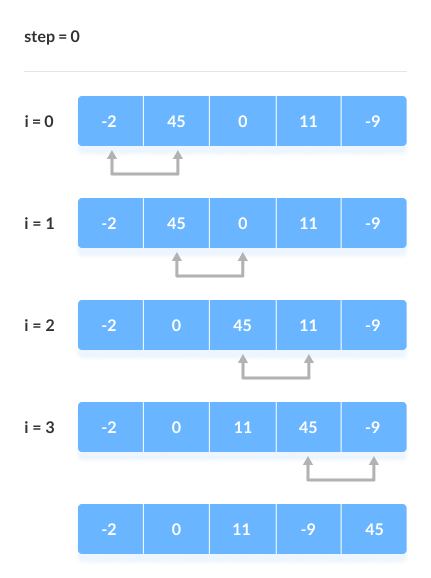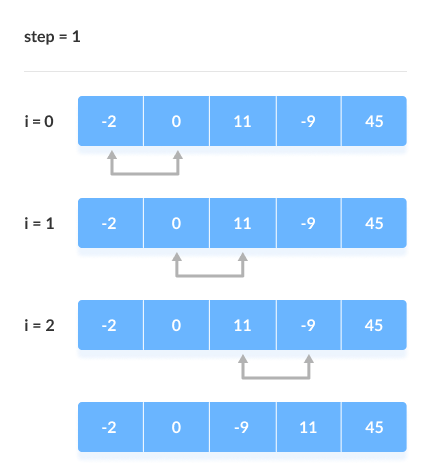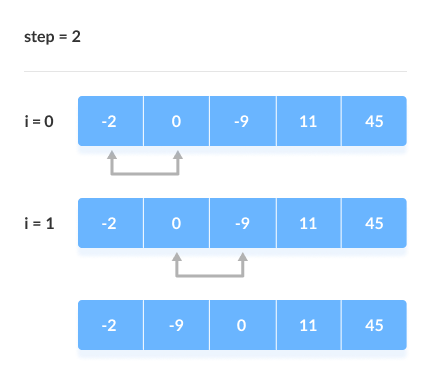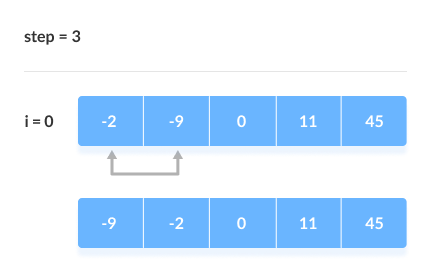Bubble Sort

Data Structures SORTING ALGORITHMS SERIESIntroduction

Bubble sort is a sorting algorithm that compares two adjacent elements and swaps them until they are not in the intended order. It is the simplest sorting algorithm.

How does Bubble Sort work?

Suppose we have an array [-2, 45, 0, 11,-9] that we are willing to sort in ascending order.

1. First Iteration or First Pass

1. Starting with index zero, compare the first and second elements.
2. If the first element is greater than the second element, swap them.
3. Compare the second and third elements, and swap them if the second element is greater than the third element.
4. Repeat the above process till the last element2. Remaining Iteration

The same process goes on for the remaining iterations. We will notice that after each iteration, the largest element among the unsorted elements is placed at the end.In each iteration, the comparison takes place up to the last unsorted element.The array is sorted when all the unsorted elements are placed at their correct positions.Algorithm

bubbleSort(array)
for i <- 1 to indexOfLastUnsortedElement-1
if leftElement > rightElement
swap leftElement and rightElement
end bubbleSort

Code Implementation

In Java

package algorithms.sorting;

import java.util.Arrays;

public class BubbleSort {
public static void main(String[] args) {
int[] nums = {-2, 45, 0, 11,-9};
BubbleSort bs = new BubbleSort();
int swaps = bs.sort(nums);
System.out.println(Arrays.toString(nums));
System.out.println("Total swaps done : " + swaps);
}

public int sort(int[] arr) {
int swaps = 0;

for (int i = 0; i < arr.length; i++) {
for (int j = 1; j < arr.length - i; j++) {
if (arr[j] < arr[j - 1]) {
int temp = arr[j];
arr[j] = arr[j - 1];
arr[j - 1] = temp;
swaps++;
}
}
}
return swaps;
}
}

In Python

def bubble_sort(array):
swaps = 0
for i in range(len(array)):
for j in range(1, len(array) - i):
if array[j] < array[j - 1]:
temp = array[j]
array[j] = array[j-1]
array[j-1] = temp
swaps += 1

return swaps

if __name__ == '__main__':
nums = [-2, 45, 0, 11, -9]
total_swaps = bubble_sort(nums)
print(nums)
print(f"Total swaps done : {total_swaps}")

Output

[-9, -2, 0, 11, 45]
Total swaps done : 6

Optimized Bubble Sort Algorithm

One thing, we can notice in the above algorithm is that even if the array is already sorted, the comparisons are still done which is useless. This increases the execution time.

To solve this, we can take an extra boolean variable swapped or isSwapped which is set to true if there occurs swapping of elements. Otherwise, it is set false

After an iteration, if there is no swapping, the value of swapped or isSwapped will be false. This means elements are already sorted and there is no need to perform further iterations.

This will reduce the execution time and helps to optimize the bubble sort.

Algorithm for Optimized Bubble Sort

bubbleSort(array)
swapped <- false
for i <- 1 to indexOfLastUnsortedElement-1
if leftElement > rightElement
swap leftElement and rightElement
swapped <- true
end bubbleSort

Code Implementation

In Java

package algorithms.sorting;

import java.util.Arrays;

public class BubbleSort {
public static void main(String[] args) {
int[] nums = {-2, 45, 0, 11,-9};
BubbleSort bs = new BubbleSort();
int swaps = bs.sort(nums);
System.out.println(Arrays.toString(nums));
System.out.println("Total swaps done : " + swaps);
}

public int sort(int[] arr) {
boolean isSwapped;
int swaps = 0;

for (int i = 0; i < arr.length; i++) {
isSwapped = false;
for (int j = 1; j < arr.length - i; j++) {
if (arr[j] < arr[j - 1]) {
int temp = arr[j];
arr[j] = arr[j - 1];
arr[j - 1] = temp;
isSwapped = true;
swaps++;
}
}
if (!isSwapped) break;
}
return swaps;
}
}

In Python

def bubble_sort(array):
swaps = 0
for i in range(len(array)):
is_swapped = False
for j in range(1, len(array) - i):
if array[j] < array[j - 1]:
temp = array[j]
array[j] = array[j-1]
array[j-1] = temp
is_swapped = True
swaps += 1

if not is_swapped:
break

return swaps

if __name__ == '__main__':
nums = [-2, 45, 0, 11, -9]
total_swaps = bubble_sort(nums)
print(nums)
print(f"Total swaps done : {total_swaps}")

Output

[-9, -2, 0, 11, 45]
Total swaps done : 6

Bubble Sort Complexity

Time Complexity Space Complexity Best O(n) Worst O(n2) Average O(n2) O(1) Yes

Detailed Complexity

Bubble Sort compares the adjacent elements.

Cycle Number of Comparisons
1st (n-1)
2nd (n-2)
3rd (n-3)
....... ......
last 1

Hence, the number of comparisons is

(n-1) + (n-2) + (n-3) +.....+ 1 = n(n-1)/2

which is nearly equal to n2

Hence, Complexity: O(n2)

Time Complexity

• Worst Case Complexity: O(n2)
If we want to sort in ascending order and the array is in descending order then the worst case occurs.
• Best Case Complexity: O(n)
If the array is already sorted, then there is no need for sorting.
• Average Case Complexity: O(n2)
It occurs when the elements of the array are in jumbled order (neither ascending nor descending).

Space Complexity

• Space complexity is O(1) because an extra variable is used for swapping.
• In the optimized bubble sort algorithm, two extra variables are used. Hence, the space complexity will be O(2).

Conclusion

In this blog, we discussed the first sorting algorithm - Bubble Sort. This blog post cannot end without thanking the person who has motivated a lot of students to learn DSA, that too free of cost - Kunal Kushwaha and Community Classroom. You can also access his free DSA playlist here.

Watch his Bubble Sort Algorithm Video here :

Image Courtesy: Programiz
Visualize algorithms easily on visualgo.net.